# NCERT Solutions class 7 Maths Chapter-12 Exercise 12.1

## NCERT Solutions class 7 Maths Chapter-12 Algebraic expressions

### Algebraic expressions  Exercise-12.1

NCERT Solutions Class-7 Maths chapter-12 Algebraic expressions  Exercise-12.1 is prepared by academic team of pw all the questions of NCERT text book are solved step by step with proper and detail solutions explaining each and every questions . For More and additional questions of CBSE class 7 maths you can go to class 7 maths sections. NCERT class 7 Maths Solutions is the best way to enhanced your mathematics skill. And pw practice worksheet & question bank will help you a lot .

### Solutions of Chapter Algebraic expressions  Exercise-12.1

Question 1:

Get the algebraic expressions in the following cases using variables, constants and arithmetic operations.

(i) Subtraction of z from y.

(ii) One-half of the sum of numbers x and y.

(iii) The number z multiplied by itself.

(iv) One-fourth of the product of numbers p and q.

(v) Numbers x and y both squared and added.

(vi) Number 5 added to three times the product of numbers m and n.

(vii) Product of number y and z subtracted from 10.

(viii) Sum of numbers a and b subtracted from their product.

(i) y - x

(ii)1/2( x+y )

(iii)Z2

(iv) 1/4( pq )

(v)x2+y2

(vi)5 + 3 (mn)

(vii) 10 -(yz)

(viii) ab-(a+b)

Question 2:

(i) Identify the terms and their factors in the following expressions.  Show the terms and factors by tree diagrams.

(a) x - 3

(b) 1 + x + x2

(c)  y - y3

(d) 5xy2 + 7x2y

(e) -ab + 2b2 - 3a2

(ii) Identify terms and factors in the expression given below:

(a) -4x + 5

(b) -4x + 5y

(c) 5y + 3y2

(d) xy + 2x2y2

(e) pq + q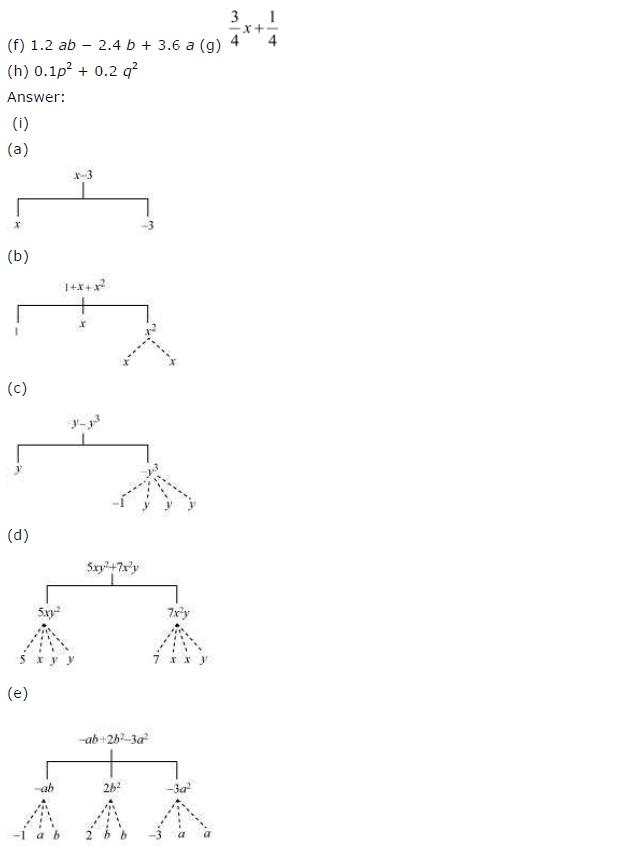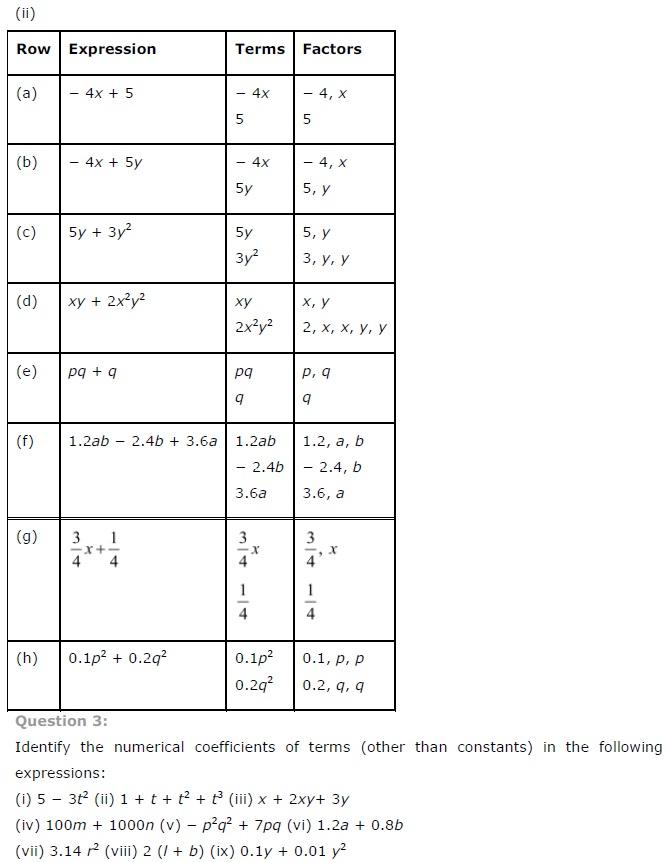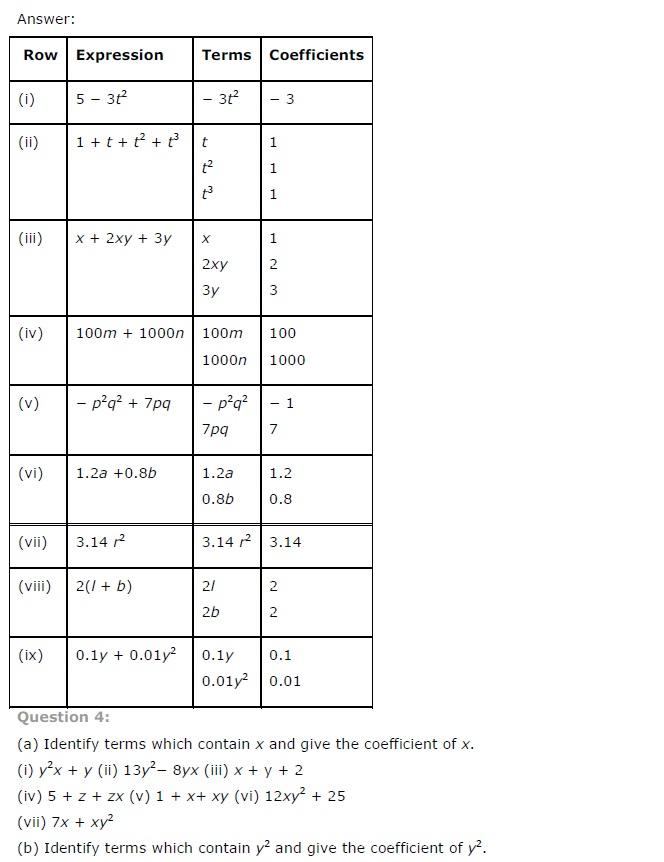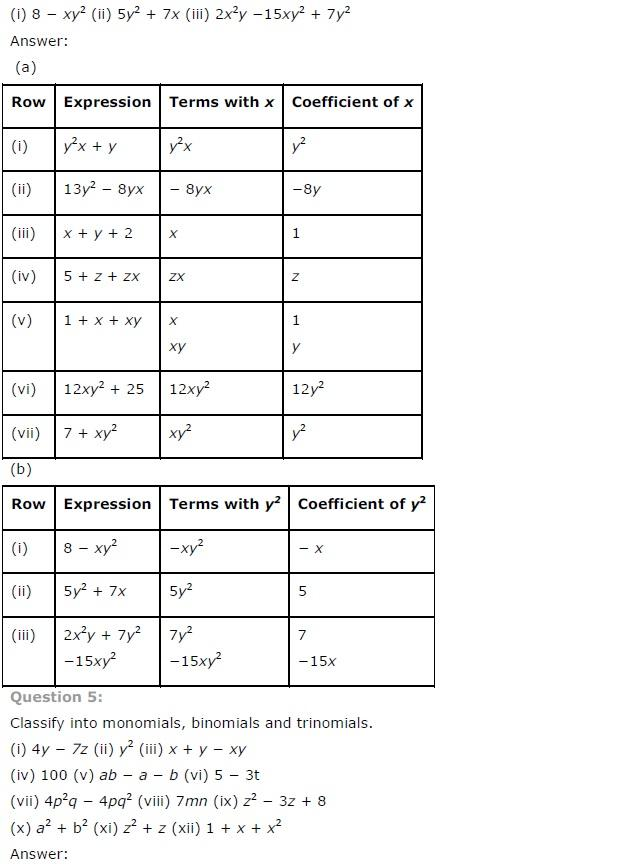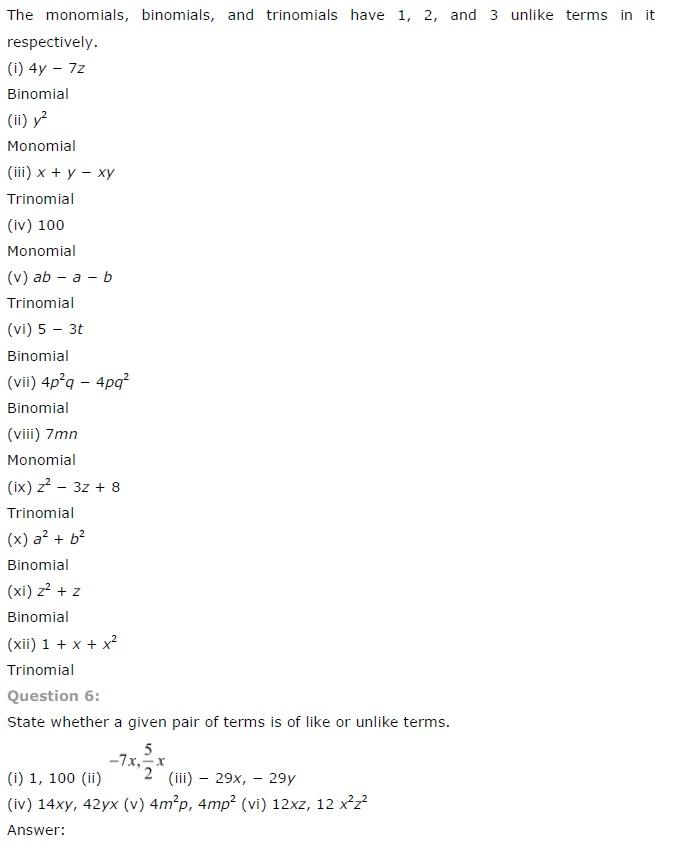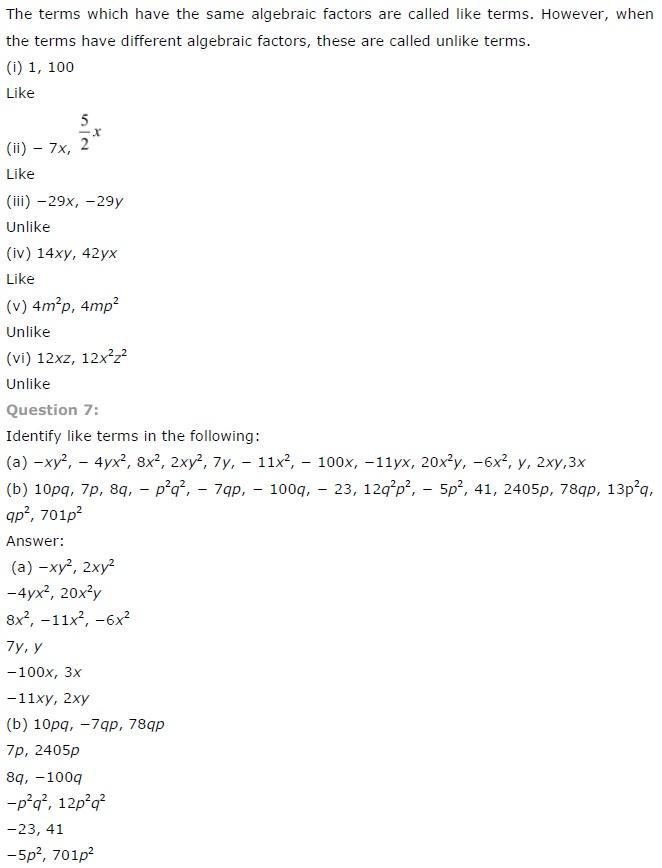NCERT CLASS 7 MATHEMATICS SOLUTIONS